Normal distribution

Here's how we get the normal probability distribution function.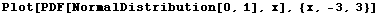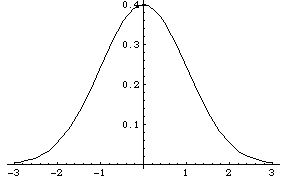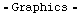The following definition returns the area (Cumulative Distribution Function) of the "standard normal distribution", i.e. a Gaussian with μ=0 and σ=1.  These are the numbers in table D-4 in the Understanding Statistics text, which is the probability for a value at or below the given z.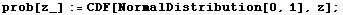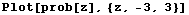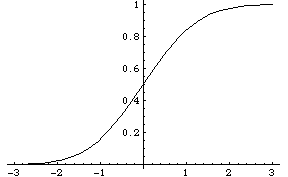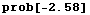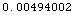Since this is in the z<0 range, its the same as the NormalPValue result.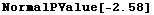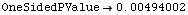But on the positive side we have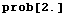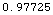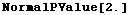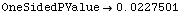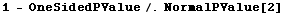To find the inverse function, Mathematica uses the Quantile property of a distribution, which returns the z value where the distribution accumulates a given total probability.  So to find out the z value such that the probability below that z is 0.9775, we do this.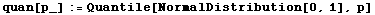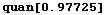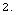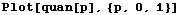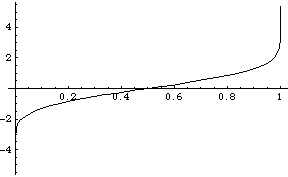Created by Mathematica  (October 2, 2003)